Next: Ideal and Perfect Bipolar Up: Bipolar Junction Transistors Previous: Basic Circuit Configurations

## Small-Signal Models

A simple transistor model is given by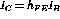. A more general model capable of describing the family of characteristic curves is given by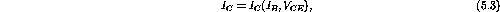where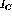is a transistor-dependent function.

For AC analysis only time changes are important and we may write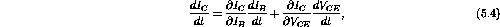where the partial derivatives are evaluated at a particular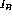and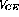- the operating point.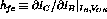is the forward current transfer ratio and describes the vertical spacing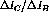between the curves. The output admittance (inverse resistance) is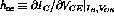and describes the slope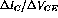of one of the curves as it passes through the operating point.

Using these definitions we may write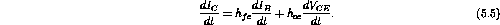The input signal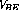is also related toand, and a similar argument to the above gives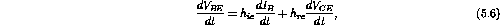where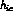is the input impedance and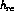is the reverse voltage ratio.

The differential equations are linear only in the limit of small AC signals, where the h parameters are effectively constant. The h parameters are in general functions of the variablesand. We arbitrarily pickedandas our independent variables. We could have picked any two of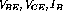and. Because the current and voltage variables are mixed the h parameters are known as hybrid parameters.

In general the current and voltage signals will have both DC and AC components. The time derivatives involve only the AC component and if we restrict ourselves to sinusoidal AC signals, we may replace the time derivatives by the signals themselves (using complex notation). Our hybrid equations become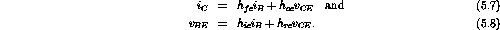The hybrid parameters are often used as the manufacturer's specification of a transistor, but there are large variations between samples. Thus one should use the actual measured parameters in any detailed calculation based on this model. Table 5.2 shows typical values for the hybrid parameters.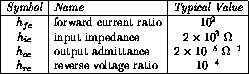Table 5.2:  Hybrid parameters.

The relationship between the voltages and currents for a transistor in the common emitter configuration is shown in figure 5.6.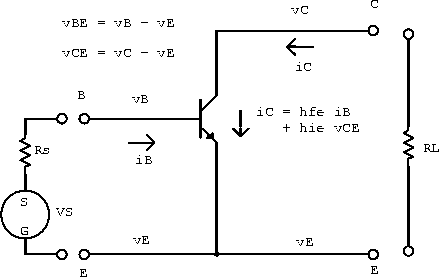Figure 5.6:  Transistor in the common emitter configuration.

We now make a few approximations to our hybrid parameter model to get an intuitive feel for how transistors behave in circuits. The voltage across the load resistor is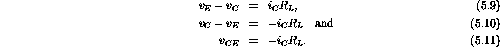Substituting this into our first hybrid equation gives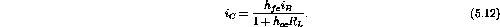If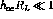(good to about 10%) we can write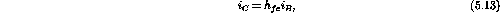which is the AC equivalent of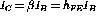. Similarly, using the second hybrid equation gives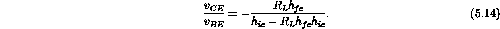If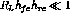(good to about 10%) we have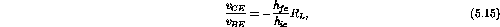which is the AC voltage gain.Next: Ideal and Perfect Bipolar Up: Bipolar Junction Transistors Previous: Basic Circuit Configurations

Doug Gingrich
Tue Jul 13 16:55:15 EDT 1999